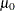### Example 57.13 Transforming to Normality

This example applies the MCMC method to the Fitness1 data set in which the variable Oxygen is transformed. Assume that Oxygen is skewed and can be transformed to normality with a logarithmic transformation. The following statements invoke the MI procedure and specify the transformation. The TRANSFORM statement specifies the log transformation for Oxygen. Note that the values displayed for Oxygen in all of the results correspond to transformed values.

proc mi data=Fitness1 seed=32937921 mu0=50 10 180 out=outex13;
transform log(Oxygen);
mcmc chain=multiple displayinit;
var Oxygen RunTime RunPulse;
run;


The Missing Data Patterns table in Output 57.13.1 lists distinct missing data patterns with corresponding statistics for the Fitness1 data. Note that the values of Oxygen shown in the tables are transformed values.

Output 57.13.1: Missing Data Patterns

The MI Procedure

Missing Data Patterns
Group Oxygen RunTime RunPulse Freq Percent Group Means
Oxygen RunTime RunPulse
1 X X X 21 67.74 3.829760 10.809524 171.666667
2 X X . 4 12.90 3.851813 10.137500 .
3 X . . 3 9.68 3.955298 . .
4 . X X 1 3.23 . 11.950000 176.000000
5 . X . 2 6.45 . 9.885000 .

The Variable Transformations table in Output 57.13.2 lists the variables that have been transformed.

Output 57.13.2: Variable Transformations

Variable Transformations
Variable _Transform_
Oxygen LOG

The Initial Parameter Estimates for MCMC table in Output 57.13.3 displays the starting mean and covariance estimates used in the MCMC method.

Output 57.13.3: Initial Parameter Estimates

Initial Parameter Estimates for MCMC
_TYPE_ _NAME_ Oxygen RunTime RunPulse
MEAN   3.846122 10.557605 171.382949
COV Oxygen 0.010827 -0.120891 -0.328772
COV RunTime -0.120891 1.744580 3.011180
COV RunPulse -0.328772 3.011180 82.747609

Output 57.13.4 displays variance information from the multiple imputation.

Output 57.13.4: Variance Information

Variance Information
Variable Variance DF Relative
Increase
in Variance
Fraction
Missing
Information
Relative
Efficiency
Between Within Total
* Oxygen 0.000016175 0.000401 0.000420 26.499 0.048454 0.047232 0.990642
RunTime 0.001762 0.065421 0.067536 27.118 0.032318 0.031780 0.993684
RunPulse 0.205979 3.116830 3.364004 25.222 0.079303 0.075967 0.985034

Output 57.13.5 displays parameter estimates from the multiple imputation. Note that the parameter value ofhas also been transformed using the logarithmic transformation.

Output 57.13.5: Parameter Estimates

Parameter Estimates
Variable Mean Std Error 95% Confidence Limits DF Minimum Maximum Mu0 t for H0:
Mean=Mu0
Pr > |t|
* Oxygen 3.845175 0.020494 3.8031 3.8873 26.499 3.838599 3.848456 3.912023 -3.26 0.0030
RunTime 10.560131 0.259876 10.0270 11.0932 27.118 10.493031 10.600498 10.000000 2.16 0.0402
RunPulse 171.802181 1.834122 168.0264 175.5779 25.222 171.251777 172.498626 180.000000 -4.47 0.0001

The following statements list the first 10 observations of the data set outex13 in Output 57.13.6. Note that the values for Oxygen are in the original scale.

proc print data=outex13(obs=10);
title 'First 10 Observations of the Imputed Data Set';
run;


Output 57.13.6: Imputed Data Set in Original Scale

 First 10 Observations of the Imputed Data Set

Obs _Imputation_ Oxygen RunTime RunPulse
1 1 44.6090 11.3700 178.000
2 1 45.3130 10.0700 185.000
3 1 54.2970 8.6500 156.000
4 1 59.5710 7.1440 167.012
5 1 49.8740 9.2200 170.092
6 1 44.8110 11.6300 176.000
7 1 38.5834 11.9500 176.000
8 1 43.7376 10.8500 158.851
9 1 39.4420 13.0800 174.000
10 1 60.0550 8.6300 170.000

Note that the results in Output 57.13.6 can also be produced from the following statements without using a TRANSFORM statement. A transformed value of log(50)=3.91202 is used in the MU0= option.

data temp;
set Fitness1;
LogOxygen= log(Oxygen);
run;
proc mi data=temp seed=14337921 mu0=3.91202 10 180 out=outtemp;
mcmc chain=multiple displayinit;
var LogOxygen RunTime RunPulse;
run;
data outex13;
set outtemp;
Oxygen= exp(LogOxygen);
run;